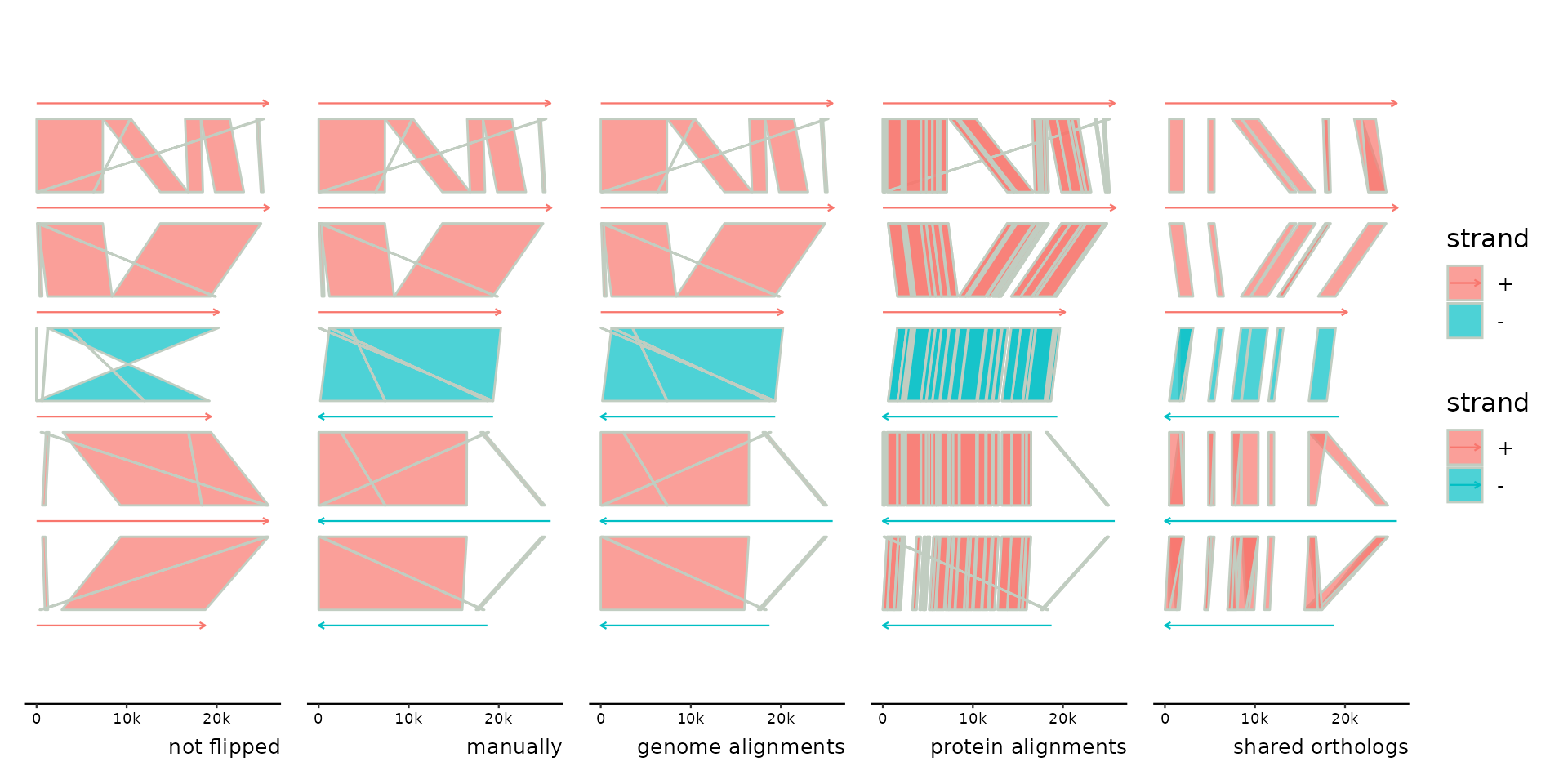flip and flip_seqs reverse-complement specified bins or individual sequences and their features. flip_by_links automatically flips bins using a heuristic that maximizes the amount of forward strand links between neighboring bins.

flip(x, ..., .bin_track = seqs)

flip_seqs(x, ..., .bins = everything(), .seq_track = seqs, .bin_track = seqs)

flip_by_links(x, link_track = 1, min_coverage = 0.2)

## Arguments

x

a gggenomes object

...

bins or sequences to flip in dplyr::select like syntax (numeric position or unquoted expressions)

.bin_track, .seq_track

when using a function as selector such as tidyselect::where(), this specifies the track in which context the function is evaluated.

.bins

preselection of bins with sequences to flip. Useful if selecting by numeric position. It sets the context for selection, for example the 11th sequences of the total set might more easily described as the 2nd sequences of the 3rd bin: flip_seqs(2, .bins=3).

the link track to use for flipping bins nicely

min_coverage

at least this much of the shorter bin must be covered by links supporting a flip to actually carry it out.

## Examples

library(patchwork)
p <- gggenomes(genes=emale_genes) +
geom_seq(aes(color=strand), arrow=TRUE) +
expand_limits(color=c("-")) +
labs(caption="not flipped")
#> No seqs provided, inferring seqs from feats

# nothing flipped

# flip manually
flip(4:6) + labs(caption="manually")

# flip automatically based on genome-genome links
#> Flipping: 4,5,6

# flip automatically based on protein-protein links
#> Transforming sublinks with "aa2nuc". Disable with .transform = "none"
#> Flipping: 4,5,6

# flip automatically based on genes linked implicitly by belonging
# to the same clusters of orthologs (or any grouping of your choice)
p4 <- p %>% add_clusters(emale_cogs) %>%
#> Joining with by = join_by(feat_id)
#> Flipping: 4,5,6

p0 + p1 + p2 + p3 + p4 + plot_layout(nrow=1, guides="collect")# flip seqs inside bins
s0 <- tibble(
bin_id = c("A", "B", "B", "B", "C", "C", "C"),
seq_id = c("a1","b1","b2","b3","c1","c2","c3"),
length = c(1e4, 6e3, 2e3, 1e3, 3e3, 3e3, 3e3))

p <- gggenomes(seqs=s0) +
geom_seq(aes(color=bin_id), size=1, arrow = arrow(angle = 30, length = unit(10, "pt"),
ends = "last", type = "open")) +
geom_bin_label() + geom_seq_label() +
expand_limits(color=c("A","B","C"))

p1 <- p %>% flip_seqs(6)
p2 <- p %>% flip_seqs(c2)
p3 <- p %>% flip_seqs(2, .bins = C)

p + p1 + p2 + p3 + plot_layout(nrow=1, guides="collect")# fancy flipping using tidyselect::where for dynamic selection
p <- gggenomes(emale_genes,emale_seqs) %>% add_clusters(emale_cogs) +
geom_seq(color="grey70", size=1, arrow = arrow(angle = 30, length = unit(15, "pt"),
ends = "last", type = "open")) +
geom_gene(aes(fill=cluster_id))
#> Joining with by = join_by(feat_id)

# flip all short seqs - where() applied to .bin_track=seqs
p1 <- p %>% flip(where(~.x$length < 21000)) # flip all seqs with MCP on "-" - where() applied to .bin_track=genes p2 <- p %>% flip(where(~any(.x$strand[.x\$cluster_id %in% "cog-MCP"] == "-")), .bin_track=genes)

p + p1 + p2 + plot_layout(nrow=1, guides="collect") & theme(legend.position = "bottom")